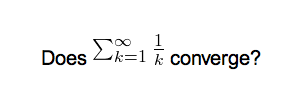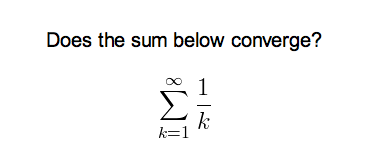# Math and Symbols

## MathJax

MathJax is a modern, browser-based typesetting system, useful for mathematical and chemical equations. It does not require the installation of any extra software, so it is easy to use, and it is recommended for most users.

MathJax is supported out of the box on Anki 2.1+, AnkiMobile, and AnkiDroid 2.9+.

To try it out:

1. Type the following in a field:

\sqrt{x}

2. Select the text you just typed.

3. Click the rightmost button in the editor, and choose "MathJax inline" from the menu. Anki will change the text so it reads:

$$\sqrt{x}$$

4. If you click the Cards… button, you’ll see a preview of how the equation will appear when the card is reviewed.

Anki’s MathJax support expects content in TeX format. If you’re not familiar with TeX formatting, please see this cheatsheet. Please note that point 2 does not apply in Anki - Anki uses $$ and $$ for inline equations, and $ and $ for display equations.

If you want to use newlines in a MathJax expression, please use Shift+Enter instead of just Enter, as a normal newline will prevent MathJax from working correctly.

Anki includes built in support for mhchem for rendering chemical equations. Please see the 'chemical equations' section and the following sections for more information: https://mhchem.github.io/MathJax-mhchem/

It is possible to customize some settings.

## LaTeX

LaTeX is a powerful typesetting system, useful for entering mathematical formulas, chemical formulas, musical notation and so on. Anki provides some support for LaTeX, allowing you to enter LaTeX code in your notes. When you review a card, Anki will call LaTeX and display the generated image instead.

LaTeX is more work to set up, and images can only be generated with the computer version of Anki - though once generated, the images can be displayed by mobile clients. Because of the extra complexity LaTeX brings, it is only recommended for users that need more features than MathJax provides.

### Assumed Knowledge

Anki’s LaTeX support is not turn-key: it is assumed that you know how to use LaTeX already, and that you have it installed. If you have no experience with LaTeX, please consult one of the many guides available on the internet. If you are having trouble with markup, please ask on a LaTeX forum.

To install LaTeX, on Windows use MiKTeX; on macOS use MacTeX, and on Linux use your distro’s package manager. Dvipng must also be installed.

On Windows, go to Settings in MikTeX’s maintenance window, and make sure "Install missing packages on the fly" is set to "Always", not to "Ask me first". If you continue to have difficulties, one user reported that running Anki as an administrator until all the packages were fetched helped.

On macOS, LaTeX has only been tested with MacTeX and BasicTeX. If you use BasicTeX, you need to install dvipng separately, with the following command:

sudo tlmgr update --self; sudo tlmgr install dvipng


The command may not be on the path, so you may need to provide the full path, e.g /usr/local/texlive/2014basic/bin/x86_64-darwin/tlmgr.

If you are not using the above LaTeX packages, you will need to use the edit LaTeX add-on to specify the full path to latex and dvipng.

### Web/Mobile

When you review a card with LaTeX on it, Anki will generate an image for that LaTeX and place the image in your collection’s media folder for future use. The web & mobile clients will display these images if they already exist, but can not generate the images on their own.

To avoid having to review all your cards at least once before you can study on the other clients, Anki can generate the images in bulk for you. To generate all the images, please go to Tools>Check Media. After that, syncing should upload the generated media to AnkiWeb and the other clients.

### Example

The most general way to input LaTeX content is to surround it with  tags. There’s a shortcut button for this documented in the editor section.

$tags must be used inside a field - placing them in the card template will cause problems. For example, entering the following on the front of an Anki flashcard: Does [latex]\begin{math}\sum_{k = 1}^{\infty}\frac{1}{k}\end{math}$ converge?


will produce this when the flashcard is viewed:The formula in the example above is called a 'text formula', because it is displayed right within the non-mathematical text. In contrast, the following example shows a 'displayed formula':

Does the sum below converge?

$\begin{displaymath}\sum_{k = 1}^{\infty}\frac{1}{k}\end{displaymath}$'Text formulas' and 'display formulas' are the most common type of LaTeX expressions, so Anki provides abbreviated versions of them. Expressions of the form:

$\begin{math}...\end{math}$


can be shortened to

[$]...[/$]


and expressions of the form

$\begin{displaymath}...\end{displaymath}$


can be shortened to

[$$]...[/$$]


For example, the two LaTeX snippets shown before are equivalent to

Does [$]\sum_{k = 1}^{\infty}\frac{1}{k}[/$] converge?


and

Does the sum below converge?

[$$]\sum_{k = 1}^{\infty}\frac{1}{k}[/$$]


respectively.

### Packages

Anki allows you to customize the LaTeX preamble so you can import custom packages for chemistry, music and so on. For example, imagine you find an example file for chemtex on the internet:

\documentclass[a4paper,12pt]{report}
\usepackage{chemtex}
\begin{document}

\initial
\begin{figure}[h]\centering
\parbox{.3\textwidth}{\ethene{H}{H$_3$C}{CH$_3$}{Br}}
\hfil
\parbox{.3\textwidth}{\cbranch{H}{S}{H}{S}{C}{S}{}{S}{H}
\xi=-200 \cright{}{Q}{C}{D}{O}{S}{OH}}
\hfil
\parbox{.3\textwidth}{\hetisix{Q}{Q}{Q}{Q}{Q}{Q}{O}{Q}{O}
\xi=-171 \fuseup{Q}{Q}{Q}{Q}{D}{Q}{D}{Q}{D}}
\caption{Chemie mit {\tt CHEMTEX}\label{a1}}
\end{figure}

\end{document}


Firstly, follow the documentation of the package and MiKTeX/MacTeX in order to install the package. To check the package is working, you’ll want to put code like the above into a .latex file and test if you can compile it from the command line. Once you’ve confirmed that the package is available and working, we can integrate it with Anki.

To use the package with Anki, click "Add" in the main window, then click the note type selection button. Click the "Manage" button, then select the note type you plan to use and click "Options". The LaTeX header and footer are shown. The header will look something like:

\documentclass[12pt]{article}
\special{papersize=3in,5in}
\usepackage{amssymb,amsmath}
\pagestyle{empty}
\setlength{\parindent}{0in}
\begin{document}


To use chemtex, you’d add the usepackage line in the earlier example, so it looks like:

\documentclass[12pt]{article}
\special{papersize=3in,5in}
\usepackage{amssymb,amsmath}
\usepackage{chemtex}
\pagestyle{empty}
\setlength{\parindent}{0in}
\begin{document}


After that, you should be able to include lines like the following in your Anki cards:

$\ethene{H}{H_3C}{CH_3}{Br}$


### Template Conflicts

As of Anki 2.1.20 / AnkiMobile 2.0.56 / AnkiDroid 2.13, this workaround is no longer required, as {{field}} text inside fields no longer causes problems. If you need to support older versions and want to keep using this syntax, please make sure you place the {{=<% %>=}} string at the very top of your front and back template, as recent Anki versions will not recognize it anywhere but the start.

For older versions:

It’s not uncommon for {{ and }} to pop up in LaTeX code when writing mathematical equations. To ensure that your LaTeX equations don’t conflict with Anki’s field replacements, it’s possible to change the separator to something else.

For example, if you have a template:

{{latex field}}


Changing it to the following will make it unlikely that the LaTeX will conflict:

{{=<% %>=}}
<%latex field%>


### Cloze Conflicts

Cloze deletions are terminated with }}, which can conflict with a }} appearing in your LaTeX. To prevent LaTeX from being interpreted as a closing cloze marker, you can put a space between any double closing braces that do not indicate the end of the cloze, so

{{c1::[$]\frac{foo}{\frac{bar}{baz}}[/$] blah blah blah.}}


will not work, but

{{c1::[$]\frac{foo}{\frac{bar}{baz} }[/$] blah blah blah.}}


will (and LaTeX ignores spaces in math mode, so your equation will render the same). If you want to avoid adding the extra space into the rendered text (for example, when you are making Cloze cards for learning programming languages), another option is to use a HTML comment when editing the card in HTML mode:

{{c1::[$]\frac{foo}{\frac{bar}{baz}<!-- -->}[/$] blah blah blah.}}


You may use either workaround if you need to use the :: character sequence within the Cloze-deleted text. The first card generated for the following note text will read [type] in C++ is a type-safe union:

{{c1::std:<!-- -->:variant::~type~}} in C++ is a {{c2::type-safe union}}


### Unsafe Commands

Anki prohibits certain commands like \input or \def from being used on cards or in templates, because allowing them could allow malicious shared decks to damage your system. (To be on the safe side, these commands are prohibited even in comments, so if you’re getting this error but don’t think you’ve used one, please double-check any comments you have in your headers, templates, and cards.) If you need to use these commands, please add them to a system package and import that package as described in the previous section.